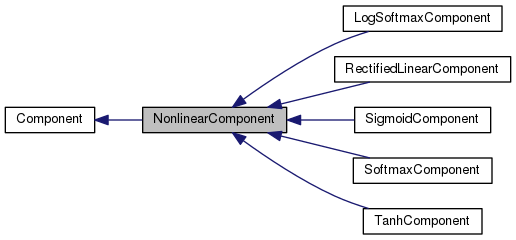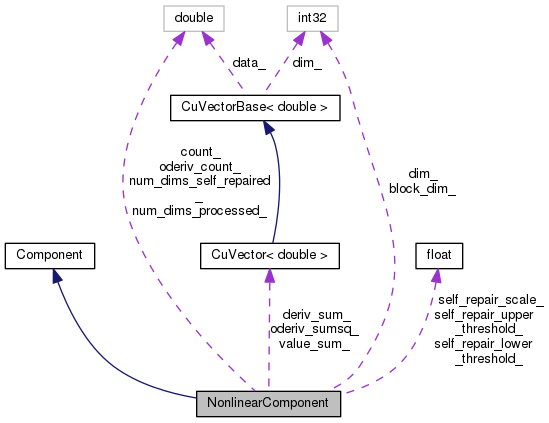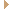NonlinearComponent Class Reference

`#include <nnet-component-itf.h>`

Inheritance diagram for NonlinearComponent:[legend]
Collaboration diagram for NonlinearComponent:[legend]

## Public Member Functions

NonlinearComponent ()

NonlinearComponent (const NonlinearComponent &other)

virtual int32 InputDim () const
Returns input-dimension of this component. More...

virtual int32 OutputDim () const
Returns output-dimension of this component. More...

virtual void InitFromConfig (ConfigLine *cfl)
Initialize, from a ConfigLine object. More...

virtual void Read (std::istream &is, bool binary)
We implement Read at this level as it just needs the Type(). More...

virtual void ZeroStats ()
Components that provide an implementation of StoreStats should also provide an implementation of ZeroStats(), to set those stats to zero. More...

virtual std::string Info () const

virtual void Write (std::ostream &os, bool binary) const
Write component to stream. More...

virtual void Scale (BaseFloat scale)
This virtual function when called on – an UpdatableComponent scales the parameters by "scale" when called by an UpdatableComponent. More...

virtual void Add (BaseFloat alpha, const Component &other)
This virtual function when called by – an UpdatableComponent adds the parameters of another updatable component, times some constant, to the current parameters. More...

virtual void ConsolidateMemory ()
This virtual function relates to memory management, and avoiding fragmentation. More...

const CuVector< double > & ValueSum () const

const CuVector< double > & DerivSum () const

double Count () constPublic Member Functions inherited from Component
virtual void * Propagate (const ComponentPrecomputedIndexes *indexes, const CuMatrixBase< BaseFloat > &in, CuMatrixBase< BaseFloat > *out) const =0
Propagate function. More...

virtual void Backprop (const std::string &debug_info, const ComponentPrecomputedIndexes *indexes, const CuMatrixBase< BaseFloat > &in_value, const CuMatrixBase< BaseFloat > &out_value, const CuMatrixBase< BaseFloat > &out_deriv, void *memo, Component *to_update, CuMatrixBase< BaseFloat > *in_deriv) const =0
Backprop function; depending on which of the arguments 'to_update' and 'in_deriv' are non-NULL, this can compute input-data derivatives and/or perform model update. More...

virtual void StoreStats (const CuMatrixBase< BaseFloat > &in_value, const CuMatrixBase< BaseFloat > &out_value, void *memo)
This function may store stats on average activation values, and for some component types, the average value of the derivative of the nonlinearity. More...

virtual void GetInputIndexes (const MiscComputationInfo &misc_info, const Index &output_index, std::vector< Index > *desired_indexes) const
This function only does something interesting for non-simple Components. More...

virtual bool IsComputable (const MiscComputationInfo &misc_info, const Index &output_index, const IndexSet &input_index_set, std::vector< Index > *used_inputs) const
This function only does something interesting for non-simple Components, and it exists to make it possible to manage optionally-required inputs. More...

virtual void ReorderIndexes (std::vector< Index > *input_indexes, std::vector< Index > *output_indexes) const
This function only does something interesting for non-simple Components. More...

virtual ComponentPrecomputedIndexesPrecomputeIndexes (const MiscComputationInfo &misc_info, const std::vector< Index > &input_indexes, const std::vector< Index > &output_indexes, bool need_backprop) const
This function must return NULL for simple Components. More...

virtual std::string Type () const =0
Returns a string such as "SigmoidComponent", describing the type of the object. More...

virtual int32 Properties () const =0
Return bitmask of the component's properties. More...

virtual ComponentCopy () const =0
Copies component (deep copy). More...

virtual void DeleteMemo (void *memo) const
This virtual function only needs to be overwritten by Components that return a non-NULL memo from their Propagate() function. More...

Component ()

virtual ~Component ()

## Protected Types

enum  { kUnsetThreshold = -1000 }

## Protected Member Functions

void StoreStatsInternal (const CuMatrixBase< BaseFloat > &out_value, const CuMatrixBase< BaseFloat > *deriv=NULL)

void StoreBackpropStats (const CuMatrixBase< BaseFloat > &out_deriv)

const NonlinearComponentoperator= (const NonlinearComponent &other)

## Protected Attributes

int32 dim_

int32 block_dim_

CuVector< double > value_sum_

CuVector< double > deriv_sum_

double count_

CuVector< double > oderiv_sumsq_

double oderiv_count_

double num_dims_self_repaired_

double num_dims_processed_

BaseFloat self_repair_lower_threshold_

BaseFloat self_repair_upper_threshold_

BaseFloat self_repair_scale_

## Friends

class SigmoidComponent

class TanhComponent

class SoftmaxComponent

class LogSoftmaxComponent

class RectifiedLinearComponentStatic Public Member Functions inherited from Component
static ComponentReadNew (std::istream &is, bool binary)
Read component from stream (works out its type). Dies on error. More...

static ComponentNewComponentOfType (const std::string &type)
Returns a new Component of the given type e.g. More...

## Detailed Description

Definition at line 613 of file nnet-component-itf.h.

## ◆ anonymous enum

 anonymous enum
protected
Enumerator
kUnsetThreshold

Definition at line 650 of file nnet-component-itf.h.

650 { kUnsetThreshold = -1000 };

## ◆ NonlinearComponent() [2/2]

 NonlinearComponent ( const NonlinearComponent & other )
explicit

Definition at line 612 of file nnet-component-itf.cc.

612  :
613  dim_(other.dim_), block_dim_(other.block_dim_),
614  value_sum_(other.value_sum_), deriv_sum_(other.deriv_sum_),
615  count_(other.count_), oderiv_sumsq_(other.oderiv_sumsq_),
616  oderiv_count_(other.oderiv_count_),
617  num_dims_self_repaired_(other.num_dims_self_repaired_),
618  num_dims_processed_(other.num_dims_processed_),
619  self_repair_lower_threshold_(other.self_repair_lower_threshold_),
620  self_repair_upper_threshold_(other.self_repair_upper_threshold_),
621  self_repair_scale_(other.self_repair_scale_) { }

## Member Function Documentation

 void Add ( BaseFloat alpha, const Component & other )
virtual

This virtual function when called by – an UpdatableComponent adds the parameters of another updatable component, times some constant, to the current parameters.

– a NonlinearComponent (or another component that stores stats, like BatchNormComponent)– it relates to adding stats. Otherwise it will normally do nothing.

Reimplemented from Component.

Definition at line 459 of file nnet-component-itf.cc.

459  {
460  const NonlinearComponent *other =
461  dynamic_cast<const NonlinearComponent*>(&other_in);
462  KALDI_ASSERT(other != NULL);
463  if (value_sum_.Dim() == 0 && other->value_sum_.Dim() != 0)
464  value_sum_.Resize(other->value_sum_.Dim());
465  if (deriv_sum_.Dim() == 0 && other->deriv_sum_.Dim() != 0)
466  deriv_sum_.Resize(other->deriv_sum_.Dim());
467  if (oderiv_sumsq_.Dim() == 0 && other->oderiv_sumsq_.Dim() != 0)
468  oderiv_sumsq_.Resize(other->oderiv_sumsq_.Dim());
469  if (other->value_sum_.Dim() != 0)
471  if (other->deriv_sum_.Dim() != 0)
473  if (other->oderiv_sumsq_.Dim() != 0)
475  count_ += alpha * other->count_;
476  oderiv_count_ += alpha * other->oderiv_count_;
477  num_dims_self_repaired_ += alpha * other->num_dims_self_repaired_;
478  num_dims_processed_ += alpha * other->num_dims_processed_;
479 }
void Resize(MatrixIndexT dim, MatrixResizeType t=kSetZero)
Allocate the memory.
Definition: cu-vector.cc:993
void AddVec(Real alpha, const CuVectorBase< Real > &vec, Real beta=1.0)
Definition: cu-vector.cc:1237
#define KALDI_ASSERT(cond)
Definition: kaldi-error.h:185
MatrixIndexT Dim() const
Dimensions.
Definition: cu-vector.h:69

## ◆ ConsolidateMemory()

 void ConsolidateMemory ( )
virtual

This virtual function relates to memory management, and avoiding fragmentation.

It is called only once per model, after we do the first minibatch of training. The default implementation does nothing, but it can be overridden by child classes, where it may re-initialize certain quantities that may possibly have been allocated during the forward pass (e.g. certain statistics; OnlineNaturalGradient objects). We use our own CPU-based allocator (see cu-allocator.h) and since it can't do paging since we're not in control of the GPU page table, fragmentation can be a problem. The allocator always tries to put things in 'low-address memory' (i.e. at smaller memory addresses) near the beginning of the block it allocated, to avoid fragmentation; but if permanent things (belonging to the model) are allocated in the forward pass, they can permanently stay in high memory. This function helps to prevent that, by re-allocating those things into low-address memory (It's important that it's called after all the temporary buffers for the forward-backward have been freed, so that there is low-address memory available)).

Reimplemented from Component.

Definition at line 636 of file nnet-component-itf.cc.

636  {
637  { CuVector<double> temp(value_sum_); value_sum_.Swap(&temp); }
638  { CuVector<double> temp(deriv_sum_); deriv_sum_.Swap(&temp); }
639  { CuVector<double> temp(oderiv_sumsq_); oderiv_sumsq_.Swap(&temp); }
640 }
void Swap(CuVector< Real > *vec)
Definition: cu-vector.cc:1019

## ◆ Count()

 double Count ( ) const
inline

Definition at line 647 of file nnet-component-itf.h.

## ◆ DerivSum()

 const CuVector& DerivSum ( ) const
inline

Definition at line 645 of file nnet-component-itf.h.

645 { return deriv_sum_; }

## ◆ Info()

 std::string Info ( ) const
virtual

Starts with the type of the component. E.g. "SigmoidComponent dim=900", although most components will have much more info.

Reimplemented from Component.

Definition at line 409 of file nnet-component-itf.cc.

409  {
410  std::stringstream stream;
411  stream << Type() << ", dim=" << dim_;
412  if (block_dim_ != dim_)
413  stream << ", block-dim=" << block_dim_;
415  stream << ", self-repair-lower-threshold=" << self_repair_lower_threshold_;
417  stream << ", self-repair-upper-threshold=" << self_repair_upper_threshold_;
418  if (self_repair_scale_ != 0.0)
419  stream << ", self-repair-scale=" << self_repair_scale_;
420  if (count_ > 0 && value_sum_.Dim() == dim_) {
421  stream << ", count=" << std::setprecision(3) << count_
422  << std::setprecision(6);
423  stream << ", self-repaired-proportion="
424  << (num_dims_processed_ > 0 ?
426  Vector<double> value_avg_dbl(value_sum_);
427  Vector<BaseFloat> value_avg(value_avg_dbl);
428  value_avg.Scale(1.0 / count_);
429  stream << ", value-avg=" << SummarizeVector(value_avg);
430  if (deriv_sum_.Dim() == dim_) {
431  Vector<double> deriv_avg(deriv_sum_);
432  deriv_avg.Scale(1.0 / count_);
433  stream << ", deriv-avg=" << SummarizeVector(deriv_avg);
434  }
435  }
436  if (oderiv_count_ > 0 && oderiv_sumsq_.Dim() == dim_) {
437  Vector<double> oderiv_rms(oderiv_sumsq_);
438  oderiv_rms.Scale(1.0 / oderiv_count_);
439  // The ApplyMin() is so that the statement after it does not fail even if we
440  // had subtracted models (e.g. in full_progress.*.log).
441  oderiv_rms.ApplyFloor(0.0);
442  oderiv_rms.ApplyPow(0.5);
443  stream << ", oderiv-rms=" << SummarizeVector(oderiv_rms)
444  << ", oderiv-count=" << oderiv_count_;
445  }
446  return stream.str();
447 }
std::string SummarizeVector(const VectorBase< float > &vec)
Returns a string that summarizes a vector fairly succintly, for printing stats in info lines...
Definition: nnet-parse.cc:111
float BaseFloat
Definition: kaldi-types.h:29
virtual std::string Type() const =0
Returns a string such as "SigmoidComponent", describing the type of the object.
MatrixIndexT Dim() const
Dimensions.
Definition: cu-vector.h:69

## ◆ InitFromConfig()

 void InitFromConfig ( ConfigLine * cfl )
virtual

Initialize, from a ConfigLine object.

Parameters
 [in] cfl A ConfigLine containing any parameters that are needed for initialization. For example: "dim=100 param-stddev=0.1"

Implements Component.

Definition at line 623 of file nnet-component-itf.cc.

623  {
624  bool ok = cfl->GetValue("dim", &dim_);
625  block_dim_ = dim_;
626  cfl->GetValue("block-dim", &block_dim_);
627  cfl->GetValue("self-repair-lower-threshold", &self_repair_lower_threshold_);
628  cfl->GetValue("self-repair-upper-threshold", &self_repair_upper_threshold_);
629  cfl->GetValue("self-repair-scale", &self_repair_scale_);
630  if (!ok || cfl->HasUnusedValues() || dim_ <= 0 ||
631  block_dim_ <= 0 || dim_ % block_dim_ != 0)
632  KALDI_ERR << "Invalid initializer for layer of type "
633  << Type() << ": \"" << cfl->WholeLine() << "\"";
634 }
#define KALDI_ERR
Definition: kaldi-error.h:147
virtual std::string Type() const =0
Returns a string such as "SigmoidComponent", describing the type of the object.

## ◆ InputDim()

 virtual int32 InputDim ( ) const
inlinevirtual

Returns input-dimension of this component.

Implements Component.

Definition at line 619 of file nnet-component-itf.h.

## ◆ operator=()

 const NonlinearComponent& operator= ( const NonlinearComponent & other )
protected

## ◆ OutputDim()

 virtual int32 OutputDim ( ) const
inlinevirtual

Returns output-dimension of this component.

Implements Component.

Definition at line 620 of file nnet-component-itf.h.

 void Read ( std::istream & is, bool binary )
virtual

We implement Read at this level as it just needs the Type().

Implements Component.

Definition at line 481 of file nnet-component-itf.cc.

481  {
482  std::ostringstream ostr_beg, ostr_end;
483  ostr_beg << "<" << Type() << ">"; // e.g. "<SigmoidComponent>"
484  ostr_end << "</" << Type() << ">"; // e.g. "</SigmoidComponent>"
485  ExpectOneOrTwoTokens(is, binary, ostr_beg.str(), "<Dim>");
487  if (PeekToken(is, binary) == 'B') {
488  ExpectToken(is, binary, "<BlockDim>");
490  } else {
491  block_dim_ = dim_;
492  }
493  ExpectToken(is, binary, "<ValueAvg>");
495  ExpectToken(is, binary, "<DerivAvg>");
497  ExpectToken(is, binary, "<Count>");
499  if (PeekToken(is, binary) == 'O') {
500  ExpectToken(is, binary, "<OderivRms>");
502  oderiv_sumsq_.ApplyPow(2.0);
503  ExpectToken(is, binary, "<OderivCount>");
505  } else {
506  oderiv_count_ = 0.0;
508  }
512
513  std::string token;
515  if (token != '<') {
516  // this should happen only rarely, in case we couldn't push back the
517  // '<' to the stream in PeekToken().
518  token = '<' + token;
519  }
520  if (token == "<NumDimsSelfRepaired>") {
523  }
524  if (token == "<NumDimsProcessed>") {
527  }
528  if (token == "<SelfRepairLowerThreshold>") {
531  }
532  if (token == "<SelfRepairUpperThreshold>") {
535  }
536  if (token == "<SelfRepairScale>") {
539  }
540  if (token != ostr_end.str()) {
541  KALDI_ERR << "Expected token " << ostr_end.str()
542  << ", got " << token;
543  }
544 }
void ReadBasicType(std::istream &is, bool binary, T *t)
ReadBasicType is the name of the read function for bool, integer types, and floating-point types...
Definition: io-funcs-inl.h:55
void ReadToken(std::istream &is, bool binary, std::string *str)
ReadToken gets the next token and puts it in str (exception on failure).
Definition: io-funcs.cc:154
void ExpectOneOrTwoTokens(std::istream &is, bool binary, const std::string &token1, const std::string &token2)
This function is like ExpectToken but for two tokens, and it will either accept token1 and then token...
Definition: text-utils.cc:536
static void ExpectToken(const std::string &token, const std::string &what_we_are_parsing, const std::string **next_token)
void Resize(MatrixIndexT dim, MatrixResizeType t=kSetZero)
Allocate the memory.
Definition: cu-vector.cc:993
#define KALDI_ERR
Definition: kaldi-error.h:147
void ApplyPow(Real power)
Definition: cu-vector.h:147
int PeekToken(std::istream &is, bool binary)
PeekToken will return the first character of the next token, or -1 if end of file.
Definition: io-funcs.cc:170
virtual std::string Type() const =0
Returns a string such as "SigmoidComponent", describing the type of the object.
I/O.
Definition: cu-vector.cc:963
void Scale(Real value)
Definition: cu-vector.cc:1216

## ◆ Scale()

 void Scale ( BaseFloat scale )
virtual

This virtual function when called on – an UpdatableComponent scales the parameters by "scale" when called by an UpdatableComponent.

– a Nonlinear component (or another component that stores stats, like BatchNormComponent)– it relates to scaling activation stats, not parameters. Otherwise it will normally do nothing.

Reimplemented from Component.

Definition at line 449 of file nnet-component-itf.cc.

449  {
450  value_sum_.Scale(scale);
451  deriv_sum_.Scale(scale);
452  oderiv_sumsq_.Scale(scale);
453  count_ *= scale;
454  oderiv_count_ *= scale;
455  num_dims_self_repaired_ *= scale;
456  num_dims_processed_ *= scale;
457 }
void Scale(Real value)
Definition: cu-vector.cc:1216

## ◆ StoreBackpropStats()

 void StoreBackpropStats ( const CuMatrixBase< BaseFloat > & out_deriv )
protected

Definition at line 377 of file nnet-component-itf.cc.

378  {
379  // Only store these stats about every 4 minibatches. Make sure to always
380  // store the stats on the very first minibatch, or it would interact badly
381  // with the ConsolidateMemory() code.
382  if (RandInt(0, 3) == 0 && oderiv_count_ != 0)
383  return;
384
385  KALDI_ASSERT(out_deriv.NumCols() == dim_);
386
387  // Check we have the correct dimensions.
388  if (oderiv_sumsq_.Dim() != dim_) {
390  oderiv_count_ = 0.0;
391  }
392  CuVector<BaseFloat> temp(dim_);
395  oderiv_count_ += out_deriv.NumRows();
396 }
void Resize(MatrixIndexT dim, MatrixResizeType t=kSetZero)
Allocate the memory.
Definition: cu-vector.cc:993
void AddVec(Real alpha, const CuVectorBase< Real > &vec, Real beta=1.0)
Definition: cu-vector.cc:1237
#define KALDI_ASSERT(cond)
Definition: kaldi-error.h:185
MatrixIndexT Dim() const
Dimensions.
Definition: cu-vector.h:69
int32 RandInt(int32 min_val, int32 max_val, struct RandomState *state)
Definition: kaldi-math.cc:95

## ◆ StoreStatsInternal()

 void StoreStatsInternal ( const CuMatrixBase< BaseFloat > & out_value, const CuMatrixBase< BaseFloat > * deriv = `NULL` )
protected

Definition at line 349 of file nnet-component-itf.cc.

351  {
352  KALDI_ASSERT(out_value.NumCols() == dim_);
353
354  // Check we have the correct dimensions.
355  if (value_sum_.Dim() != dim_ ||
356  (deriv != NULL && deriv_sum_.Dim() != dim_)) {
357  if (value_sum_.Dim() != dim_) {
359  count_ = 0.0;
360  }
361  if (deriv != NULL && deriv_sum_.Dim() != dim_) {
363  count_ = 0.0;
365  }
366  }
367  count_ += out_value.NumRows();
368  CuVector<BaseFloat> temp(dim_);
371  if (deriv != NULL) {
374  }
375 }
void SetZero()
Math operations.
Definition: cu-vector.cc:1098
void Resize(MatrixIndexT dim, MatrixResizeType t=kSetZero)
Allocate the memory.
Definition: cu-vector.cc:993
void AddVec(Real alpha, const CuVectorBase< Real > &vec, Real beta=1.0)
Definition: cu-vector.cc:1237
#define KALDI_ASSERT(cond)
Definition: kaldi-error.h:185
MatrixIndexT Dim() const
Dimensions.
Definition: cu-vector.h:69

## ◆ ValueSum()

 const CuVector& ValueSum ( ) const
inline

Definition at line 644 of file nnet-component-itf.h.

644 { return value_sum_; }

## ◆ Write()

 void Write ( std::ostream & os, bool binary ) const
virtual

Write component to stream.

Implements Component.

Definition at line 546 of file nnet-component-itf.cc.

546  {
547  std::ostringstream ostr_beg, ostr_end;
548  ostr_beg << "<" << Type() << ">"; // e.g. "<SigmoidComponent>"
549  ostr_end << "</" << Type() << ">"; // e.g. "</SigmoidComponent>"
550  WriteToken(os, binary, ostr_beg.str());
551  WriteToken(os, binary, "<Dim>");
552  WriteBasicType(os, binary, dim_);
553  if (block_dim_ != dim_) {
554  WriteToken(os, binary, "<BlockDim>");
555  WriteBasicType(os, binary, block_dim_);
556  }
557  // Write the values and derivatives in a count-normalized way, for
558  // greater readability in text form.
559  WriteToken(os, binary, "<ValueAvg>");
560  Vector<BaseFloat> temp(value_sum_);
561  if (count_ != 0.0) temp.Scale(1.0 / count_);
562  temp.Write(os, binary);
563
564  WriteToken(os, binary, "<DerivAvg>");
565  temp.Resize(deriv_sum_.Dim());
566  temp.CopyFromVec(deriv_sum_);
567  if (count_ != 0.0) temp.Scale(1.0 / count_);
568  temp.Write(os, binary);
569
570  WriteToken(os, binary, "<Count>");
571  WriteBasicType(os, binary, count_);
572
573  WriteToken(os, binary, "<OderivRms>");
574  temp.Resize(oderiv_sumsq_.Dim());
575  temp.CopyFromVec(oderiv_sumsq_);
576  if (oderiv_count_ != 0.0) temp.Scale(1.0 / oderiv_count_);
577  // The ApplyMin() is so that the statement after it does not fail even if we
578  // had subtracted models (e.g. in full_progress.*.log).
579  temp.ApplyFloor(0.0);
580  temp.ApplyPow(0.5);
581  temp.Write(os, binary);
582
583  WriteToken(os, binary, "<OderivCount>");
584  WriteBasicType(os, binary, oderiv_count_);
585
586  WriteToken(os, binary, "<NumDimsSelfRepaired>");
588  WriteToken(os, binary, "<NumDimsProcessed>");
589  WriteBasicType(os, binary, num_dims_processed_);
591  WriteToken(os, binary, "<SelfRepairLowerThreshold>");
593  }
595  WriteToken(os, binary, "<SelfRepairUpperThreshold>");
597  }
598  if (self_repair_scale_ != 0.0) {
599  WriteToken(os, binary, "<SelfRepairScale>");
600  WriteBasicType(os, binary, self_repair_scale_);
601  }
602  WriteToken(os, binary, ostr_end.str());
603 }
void WriteToken(std::ostream &os, bool binary, const char *token)
The WriteToken functions are for writing nonempty sequences of non-space characters.
Definition: io-funcs.cc:134
virtual std::string Type() const =0
Returns a string such as "SigmoidComponent", describing the type of the object.
void WriteBasicType(std::ostream &os, bool binary, T t)
WriteBasicType is the name of the write function for bool, integer types, and floating-point types...
Definition: io-funcs-inl.h:34
MatrixIndexT Dim() const
Dimensions.
Definition: cu-vector.h:69

## ◆ ZeroStats()

 void ZeroStats ( )
virtual

Components that provide an implementation of StoreStats should also provide an implementation of ZeroStats(), to set those stats to zero.

Other components that store other types of statistics (e.g. regarding gradient clipping) should implement ZeroStats() also.

Reimplemented from Component.

Definition at line 399 of file nnet-component-itf.cc.

## ◆ LogSoftmaxComponent

 friend class LogSoftmaxComponent
friend

Definition at line 655 of file nnet-component-itf.h.

## ◆ RectifiedLinearComponent

 friend class RectifiedLinearComponent
friend

Definition at line 656 of file nnet-component-itf.h.

## ◆ SigmoidComponent

 friend class SigmoidComponent
friend

Definition at line 652 of file nnet-component-itf.h.

## ◆ SoftmaxComponent

 friend class SoftmaxComponent
friend

Definition at line 654 of file nnet-component-itf.h.

## ◆ TanhComponent

 friend class TanhComponent
friend

Definition at line 653 of file nnet-component-itf.h.

## ◆ block_dim_

 int32 block_dim_
protected

Definition at line 678 of file nnet-component-itf.h.

Referenced by NonlinearComponent::InitFromConfig().

## ◆ count_

 double count_
protected

Definition at line 684 of file nnet-component-itf.h.

## ◆ deriv_sum_

 CuVector deriv_sum_
protected

## ◆ dim_

 int32 dim_
protected

Definition at line 673 of file nnet-component-itf.h.

Referenced by NonlinearComponent::InitFromConfig().

## ◆ num_dims_processed_

 double num_dims_processed_
protected

Definition at line 695 of file nnet-component-itf.h.

## ◆ num_dims_self_repaired_

 double num_dims_self_repaired_
protected

Definition at line 694 of file nnet-component-itf.h.

## ◆ oderiv_count_

 double oderiv_count_
protected

Definition at line 691 of file nnet-component-itf.h.

## ◆ oderiv_sumsq_

 CuVector oderiv_sumsq_
protected

## ◆ self_repair_lower_threshold_

 BaseFloat self_repair_lower_threshold_
protected

Definition at line 698 of file nnet-component-itf.h.

Referenced by NonlinearComponent::InitFromConfig().

## ◆ self_repair_scale_

 BaseFloat self_repair_scale_
protected

Definition at line 700 of file nnet-component-itf.h.

Referenced by NonlinearComponent::InitFromConfig().

## ◆ self_repair_upper_threshold_

 BaseFloat self_repair_upper_threshold_
protected

Definition at line 699 of file nnet-component-itf.h.

Referenced by NonlinearComponent::InitFromConfig().

## ◆ value_sum_

 CuVector value_sum_
protected

The documentation for this class was generated from the following files: